# Formula with spatial quantities

## Definition

A formula with spatial quantities is an expression that allows handling space quantities in different contexts – physics, circuit, results post-processing.

## Use

A formula with spatial quantities is used to define:

• another space quantity
• the modulus of flux density, …

## Evaluation

The formula is evaluated during the use of the space quantity.

## Example

The example below shows the use of a formula in the context of results post-processing for a magnetic application.

In a Magneto Static application the flux density is a vector quantity: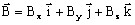,

where Bx , By , Bz are the three components of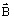on the axis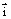,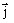and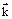.

In this example we are interested in the By component of flux density.

The spatial quantity (BY) can be expressed as function of other spatial quantities (B) by using the following formula:

BY = Comp(2,B)

The function Comp(i,V) returns the i component of the vector V.